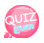# return statement

Use the return statement to return the calculated value to the function caller within the function.
What do you mean?
Functions are designed to end life after encountering a return statement and returning a specific value.
Let's look at the code we used earlier.

```<php
function plus(\$num, \$num2) {
echo \$num + \$num2;
}
?>```

In the code above, the sum of the two numbers is summed up and output through the echo statement.
And nothing.
Using it like this is the wrong way and you need to pass the sum to the part that called the function.
The return statement is responsible for returning a value.
Using the return statement in the code above, an example would be:

```    function plus(\$num, \$num2) {
return \$num + \$num2;
}
?>```

When used as above, the life of the function ends after passing the value to the part that called the function.

So let's run the code

```<?php
function plus(\$num, \$num2) {
return \$num + \$num2;
}

plus(100,200);
?>```

Running the code above does not produce any results. The value was returned, but because no echo statement was used. If you use echo before the function call ...

```<?php
function plus(\$num, \$num2) {
return \$num + \$num2;
}

echo plus(100,200);
?>```

When you run the code above, the returned value is printed by the echo statement.
Note that the function terminates when the return statement is encountered, so the code after return will not work.

```<?php
function plus(\$num, \$num2) {
return \$num + \$num2;
echo "I don't work because there's a return statement above.";
}

echo plus(100,200);
?>```

The code above shows that the echo statement inside the function did not work.
This is because the function terminated right above.